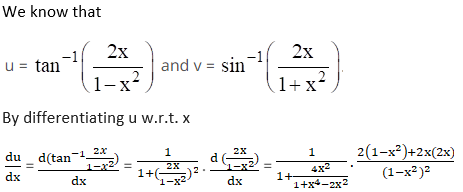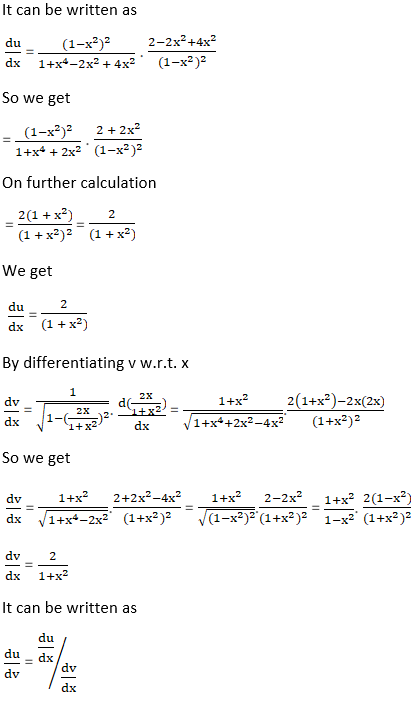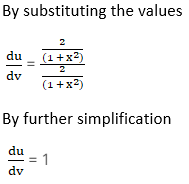# RS Aggarwal Solutions for Class 12 Maths Chapter 10: Differentiation Exercise 10H

This exercise explains students about the derivative of one function with respect to another function. Students can self analyse their knowledge about the concepts by solving problems using solutions prepared by teachers at BYJU’S. This mainly improves the problem solving and logical thinking abilities among students, which are important from the exam point of view. RS Aggarwal Solutions Class 12 Maths Chapter 10 Differentiation Exercise 10H PDF are available here.

## RS Aggarwal Solutions for Class 12 Maths Chapter 10: Differentiation Exercise 10H Download PDF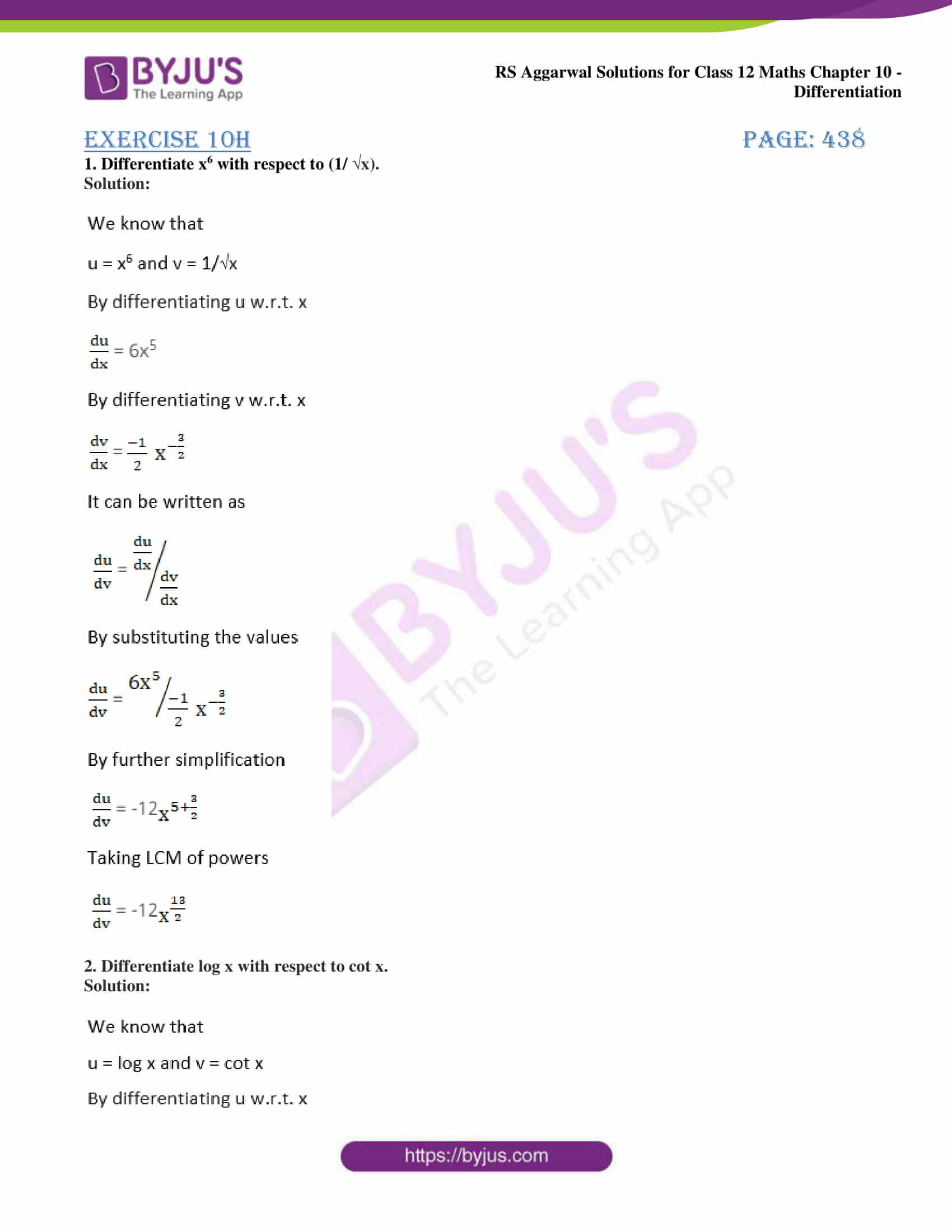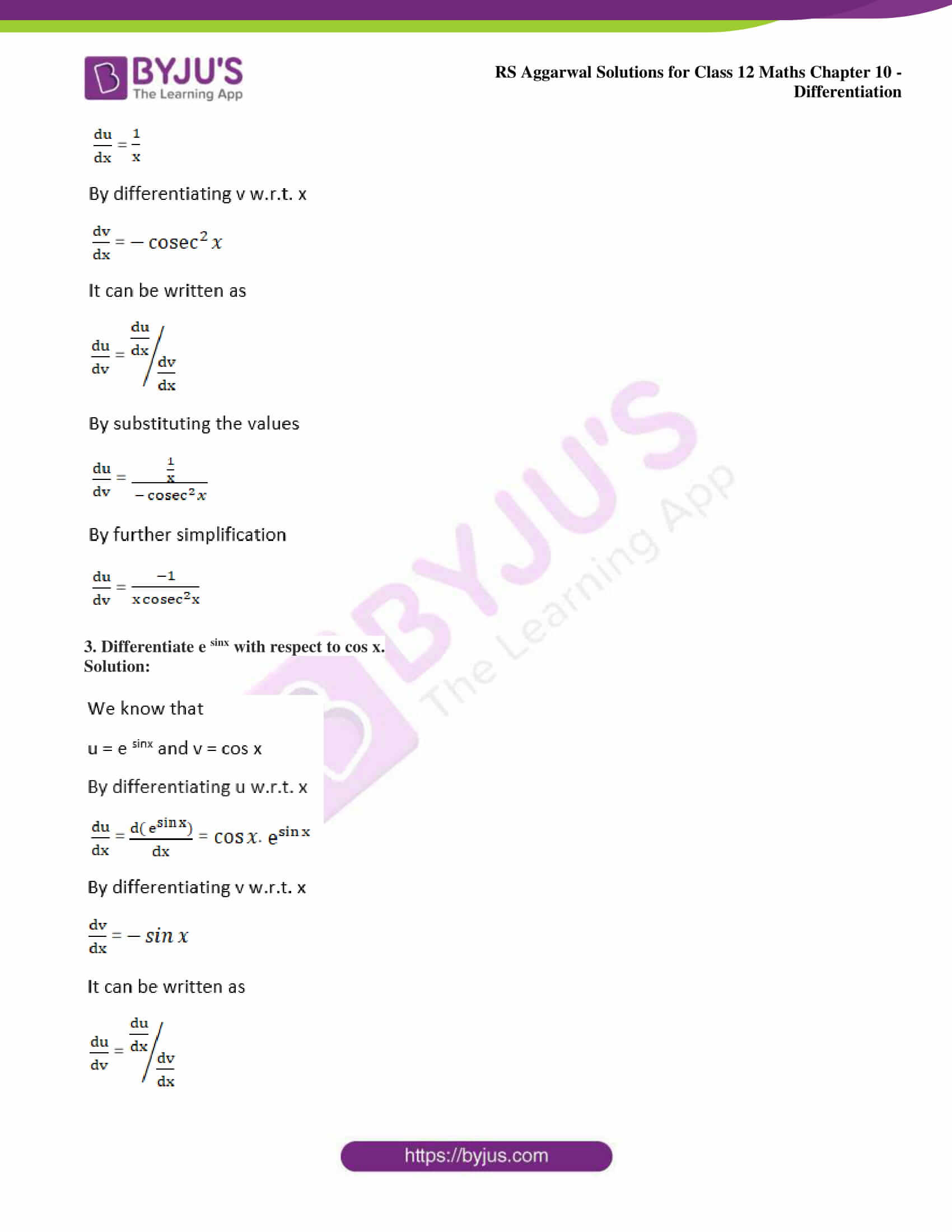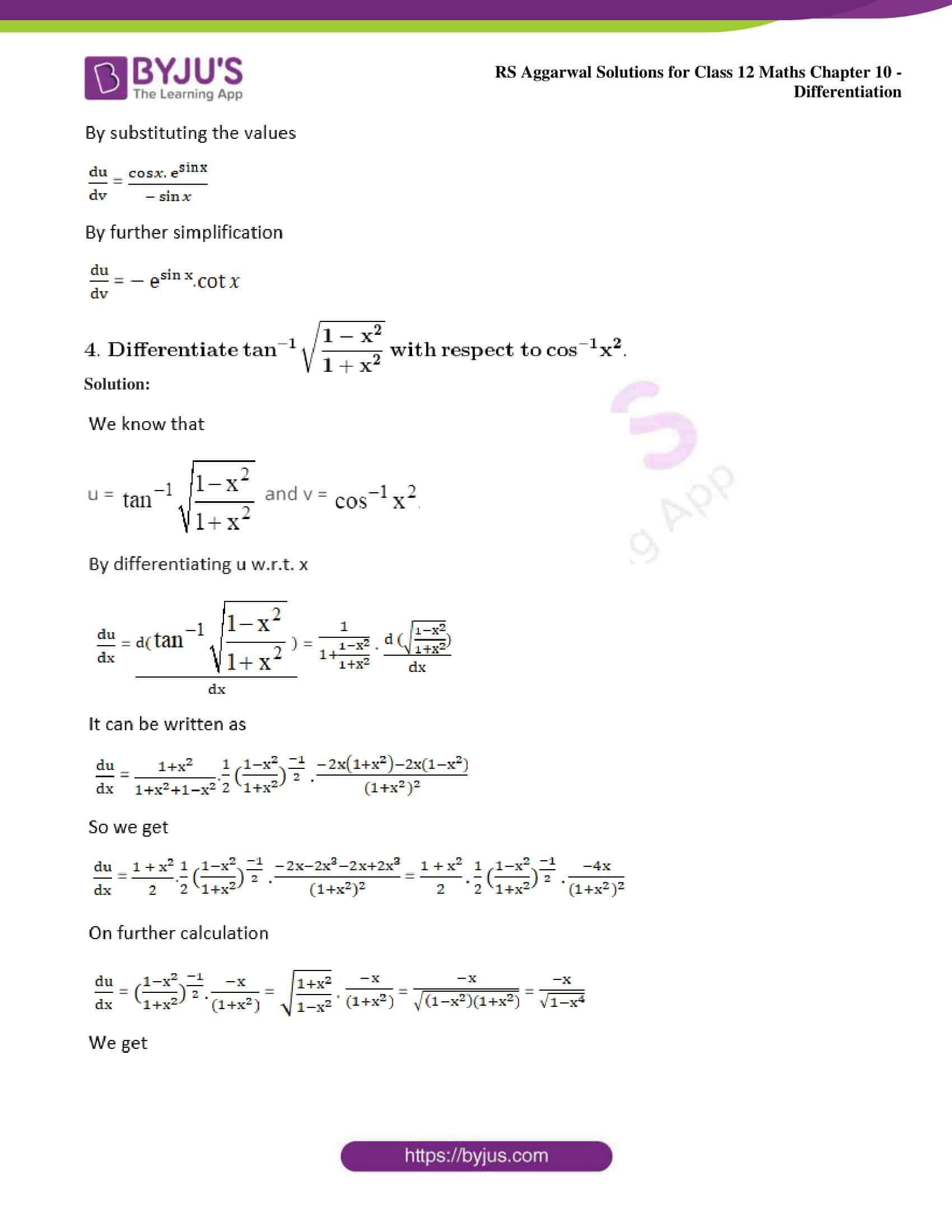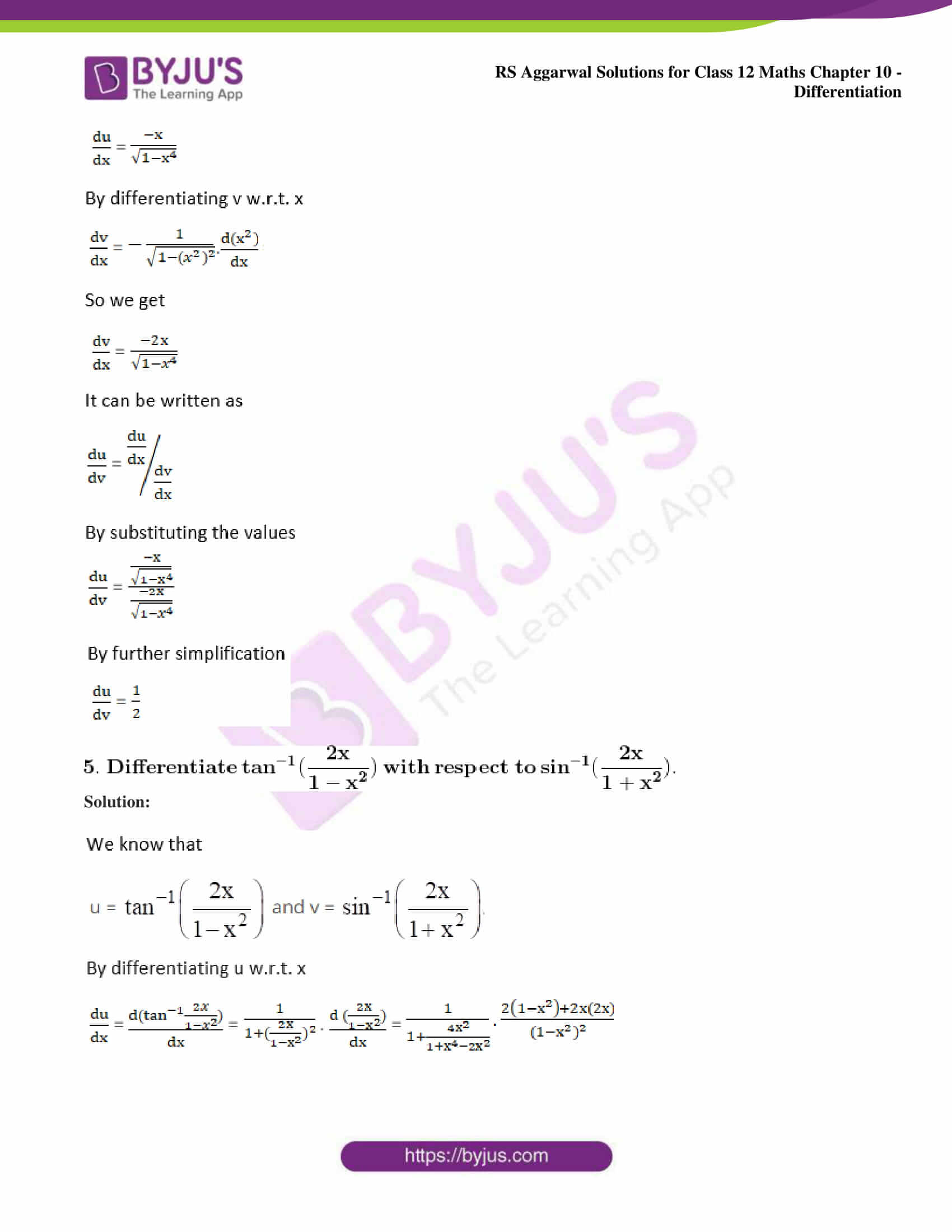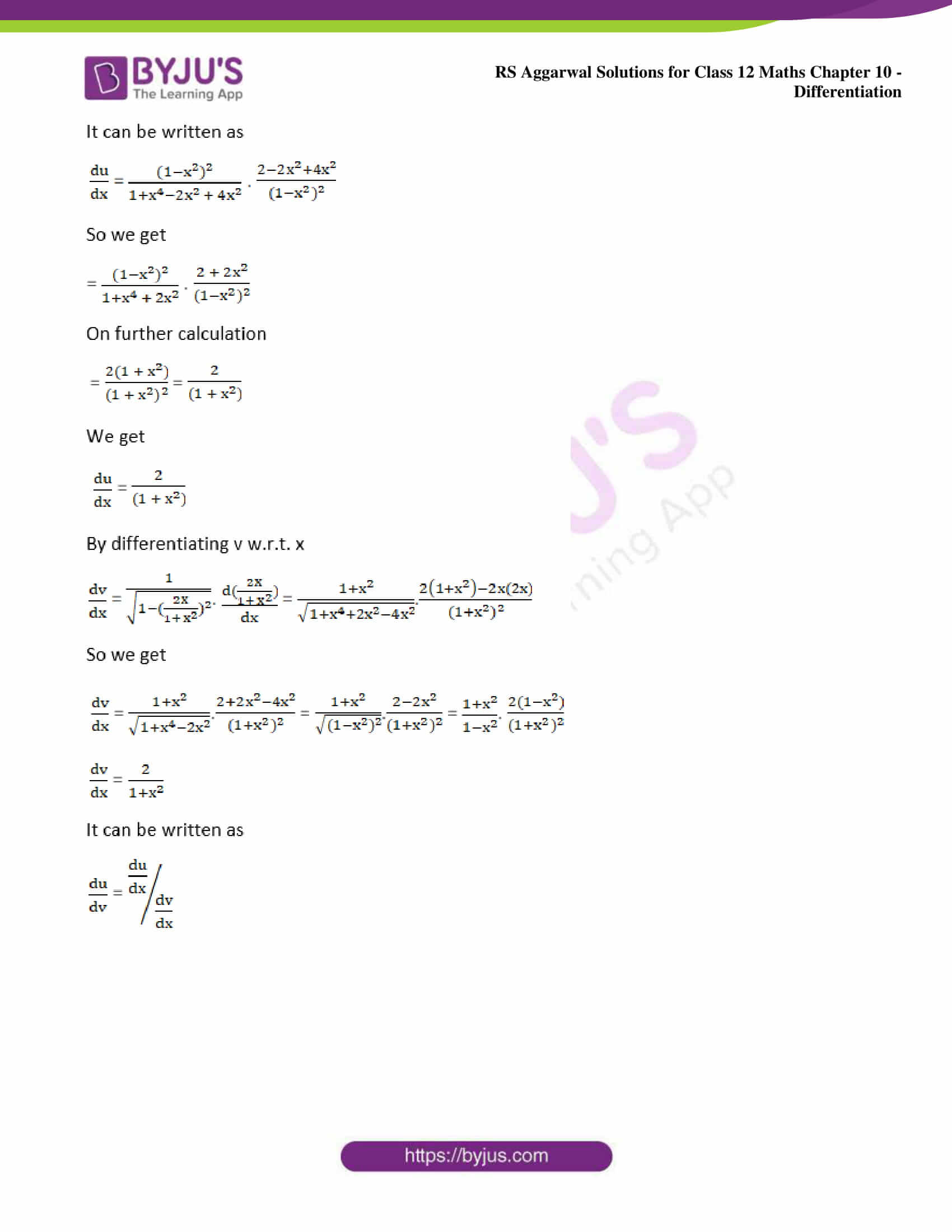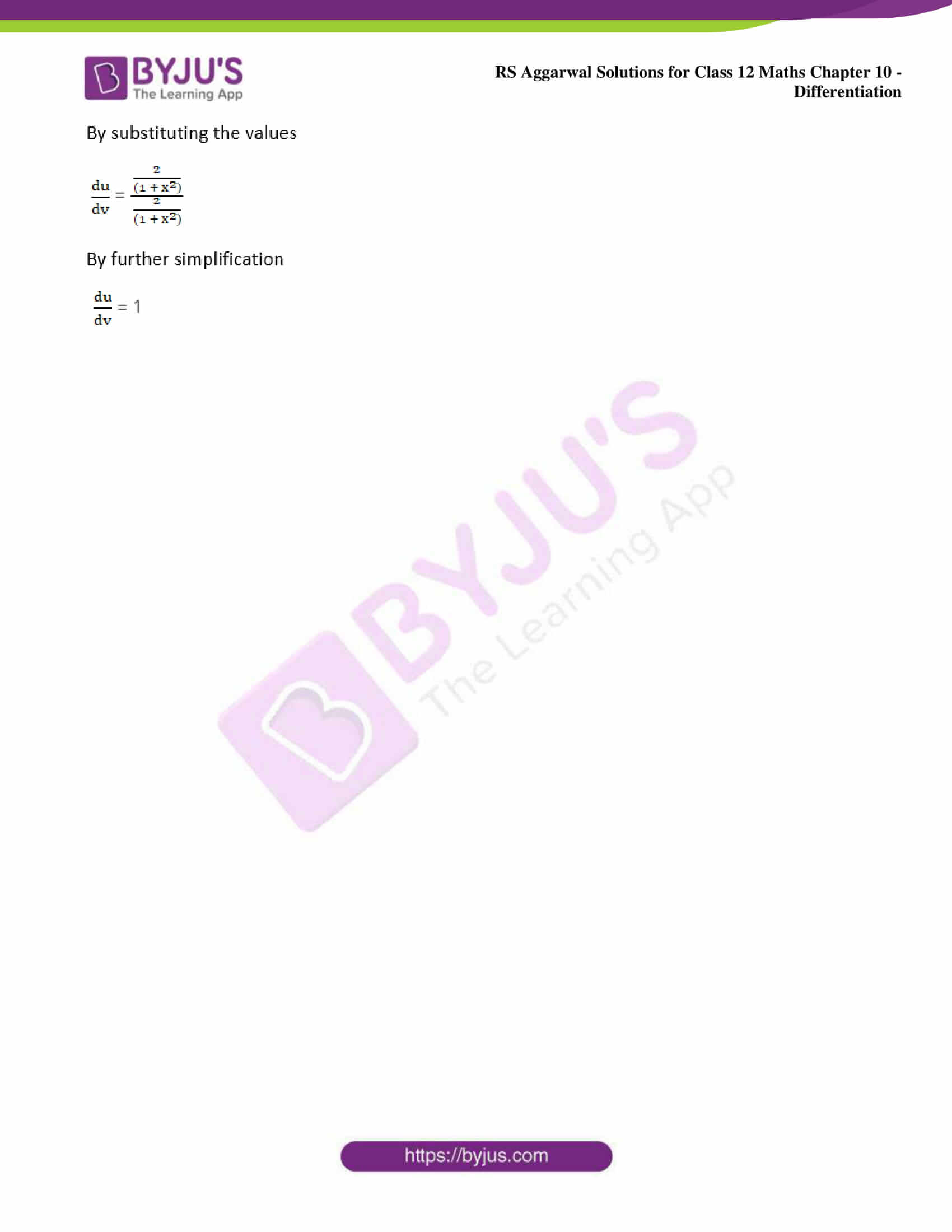### Access other exercise solutions of Class 12 Maths Chapter 10: Differentiation

Exercise 10A Solutions

Exercise 10B Solutions

Exercise 10C Solutions

Exercise 10D Solutions

Exercise 10E Solutions

Exercise 10F Solutions

Exercise 10G Solutions

Exercise 10I Solutions

Exercise 10J Solutions

### Access RS Aggarwal Solutions for Class 12 Maths Chapter 10: Differentiation Exercise 10H

1. Differentiate x6 with respect to (1/ √x).

Solution: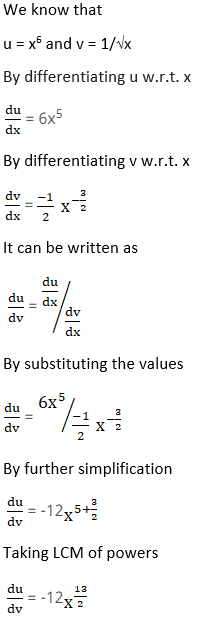2. Differentiate log x with respect to cot x.

Solution: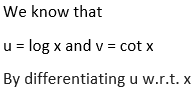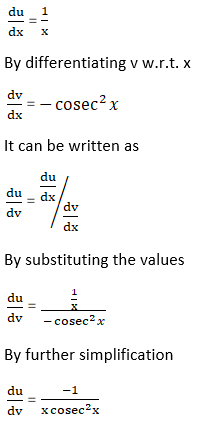3. Differentiate e sinx with respect to cos x.

Solution: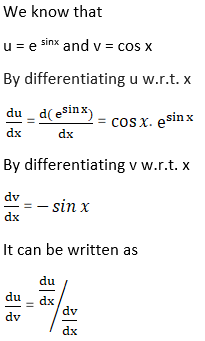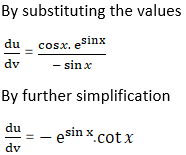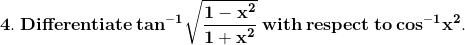Solution: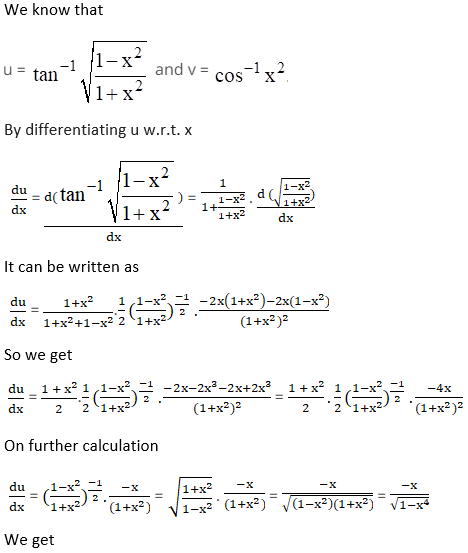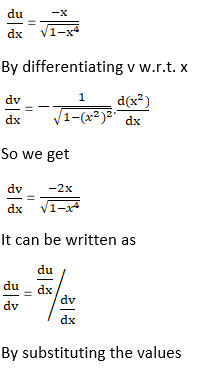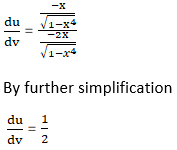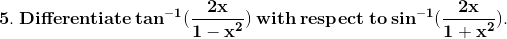Solution: